Next: B. The Historical Development Up: A. Units of Measure, Previous: A.1 Angular Quantities   Contents

# A.2 Photometric Quantities

The nomenclature of photometric quantitities in use in astronomical literature is far from standard and sometimes ambiguous. Here we therefore give a brief summary of the definitions and units of measure of these quantities as they are used in this study.
• The Luminosity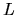of a source is the energy radiated by the whole surface of the source per unit time, that is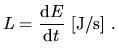(A.1)

• The Brightness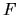of a source is the energy radiated by the whole surface of the source per unit time per unit area (of the receiver), that is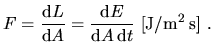(A.2)

• Most galaxies, unlike most stars, are resolved objects, so that in addition to measuring their total energy flux, we can in principle measure the energy flux per unit solid angle of the source coming from different regions. The Surface Brightness of a region of a diffuse source is the energy radiated by the region per unit time, per unit area (of the receiver) and per unit solid angle (of the source), that is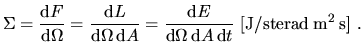(A.3)

Astronomers, however, generally express brightness and surface brightness in logarithmic units, i.e. in magnitudes (mag) and magnitudes per square second of arc (mag/arcsec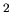), respectively. To define a magnitude scale, one has to arbitrarily choose a reference brightness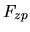, and the corresponding reference surface brightness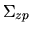ofper square second of arc. The brightness of a source expressed in magnitudes is then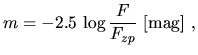(A.4)

while the surface brightness of a region of a diffuse source in magnitudes per square second of arc is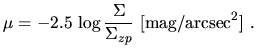(A.5)is called the zero-point of the adopted magnitude scale since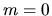for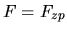(and thus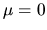for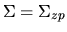).

Note that these definitions equally apply to bolometric measurements and to measurements in a given photometric band.

Note also that the sky background is often expressed in different units, such as those described by [Leinert et al. 1998].Next: B. The Historical Development Up: A. Units of Measure, Previous: A.1 Angular Quantities   Contents
Mattia Vaccari 2000-12-05